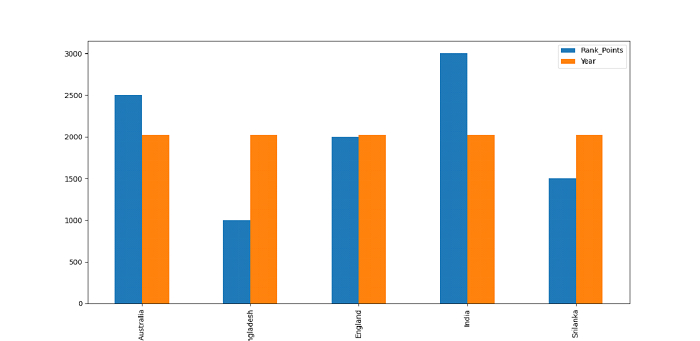# Python Pandas - Plot multiple data columns in a DataFrame?

To plot multiple columns, we will be plotting a Bar Graph. Use the plot() method and set the kind parameter to bar for Bar Graph. Let us first import the required libraries −

import pandas as pd
import matplotlib.pyplot as mp

Following is our data with Team Records −

data = [["Australia", 2500, 2021],["Bangladesh", 1000, 2021],["England", 2000, 2021],["India", 3000, 2021],["Srilanka", 1500, 2021]]

Set the data as Pandas DataFrame and add columns −

dataFrame = pd.DataFrame(data, columns=["Team","Rank_Points", "Year"])


Plot multiple columns in a bar graph. We have set the “kind” parameter as “bar” for this −

dataFrame.plot(x="Team", y=["Rank_Points","Year" ], kind="bar", figsize=(10, 9))

## Example

Following is the code −

import pandas as pd
import matplotlib.pyplot as mp

# our data
data = [["Australia", 2500, 2021],["Bangladesh", 1000, 2021],["England", 2000, 2021],["India", 3000, 2021],["Srilanka", 1500, 2021]]

# dataframe
dataFrame = pd.DataFrame(data, columns=["Team","Rank_Points", "Year"])

# plotting multiple columns in a bar Graph
dataFrame.plot(x="Team", y=["Rank_Points","Year" ], kind="bar", figsize=(10, 9))

# displaying bar graph
mp.show()

## Output

This will produce the following output −